# Cosine

(diff) ← Older revision | Latest revision (diff) | Newer revision → (diff)

One of the trigonometric functions:Its domain of definition is the entire real line; its range of values is the closed interval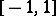; the cosine is an even periodic function (with period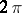). The cosine and the sine are related via the formula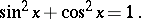The cosine and the secant are related via the formula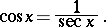The derivative of the cosine is: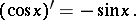The integral of the cosine is: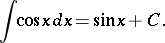The series expansion is:The inverse function is the arccosine.

The cosine and sine of a complex argumentare related to the exponential function by Euler's formula: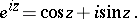Ifis a real number, then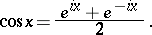If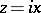(a purely imaginary number), then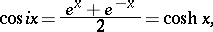where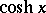is the hyperbolic cosine.

A geometric interpretation of the cosine of an argument (angle)is as follows. Consider the unit circlein the (complex) plane with origin. Letdenote the angle between the radius (thought of as varying) and the positive-axis. Thenis equal to the (signed) distance from the point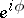oncorresponding toto the-axis. See also Sine.